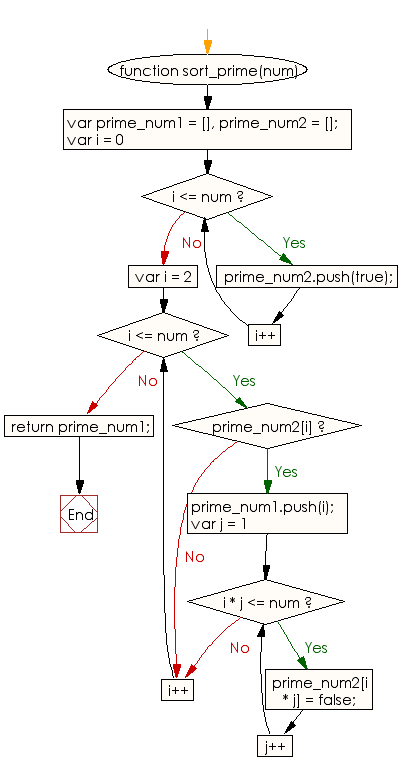# JavaScript: Sort an array of all prime numbers between 1 and a given integer

## JavaScript Basic: Exercise-109 with Solution

Write a JavaScript program to sort an array of all prime numbers between 1 and a given integer.

Sample Solution:

HTML Code:

``````<!DOCTYPE html>
<html>
<meta charset="utf-8">
<meta name="viewport" content="width=device-width">
<title> Sort an array of all prime numbers between 1 and a given integer</title>
<body>

</body>
</html>
```
```

JavaScript Code:

``````function sort_prime(num) {

var prime_num1 = [],
prime_num2 = [];
for (var i = 0; i <= num; i++) {
prime_num2.push(true);
}
for (var i = 2; i <= num; i++) {
if (prime_num2[i]) {
prime_num1.push(i);
for (var j = 1; i * j <= num; j++) {
prime_num2[i * j] = false;
}
}
}

return prime_num1;
}

console.log(sort_prime(5))
console.log(sort_prime(11))
console.log(sort_prime(19))
``````

Sample Output:

```[2,3,5]
[2,3,5,7,11]
[2,3,5,7,11,13,17,19]
```

Flowchart:ES6 Version:

``````function sort_prime(num) {
const prime_num1 = [];
const prime_num2 = [];
for (var i = 0; i <= num; i++) {
prime_num2.push(true);
}
for (var i = 2; i <= num; i++) {
if (prime_num2[i]) {
prime_num1.push(i);
for (let j = 1; i * j <= num; j++) {
prime_num2[i * j] = false;
}
}
}

return prime_num1;
}

console.log(sort_prime(5))
console.log(sort_prime(11))
console.log(sort_prime(19))
``````

Live Demo:

See the Pen javascript-basic-exercise-109 by w3resource (@w3resource) on CodePen.

What is the difficulty level of this exercise?

Test your Programming skills with w3resource's quiz.

﻿

## JavaScript: Tips of the Day

Checks if a string is an anagram of another string (case-insensitive, ignores spaces, punctuation and special characters)

Example:

```const isAnagram = (str1, str2) => {
const normalize = str =>
str
.toLowerCase()
.replace(/[^a-z0-9]/gi, '')
.split('')
.sort()
.join('');
return normalize(str1) === normalize(str2);
};
console.log(isAnagram('iceman', 'cinema')); // true
```

Output:

```true
```